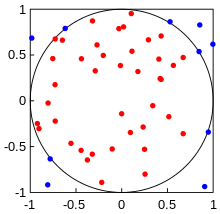# Calculating Value of Pi using Monte Carlo Technique – C PROGRAM

Recently in my Numerical Techniques class I learnt a Monte Carlo technique to calculate the value of Pi$\pi$. The procedure is really intuitive and based on probabilities and random number generation. I have already written a lot about random number generation in my recent posts.

So here’s what we do.

We consider a square extending from x=-1 to x=1 and y=-1 to y=1. That is each side is 2 units long. Now we inscribe a circle of radius 1 unit inside this square, such that the centre of the circle and the square both are at origin. Now, let’s say you drop pins/needles/rice grains or any other thing on the square randomly.The process of dropping the pins should be completely random and all positions for the landing of the pin should be equally probable. If this is the case, then we can say that the number of pins falling inside the circle (Nc) divided by the total no. of pins dropped on the square (Nt) is given by:$\frac{N_c}{N_t}=\frac{Area_{circle}}{Area_{square}}$

That is the probability of the pin falling inside the circle is directly proportional to the area of the circle. I hope this step is intuitive enough for you.

Well, that’s it. The above relation basically gives you the value of Pi. How?

Well, the area of circle in our case is just$Area_{circle}=\pi$ (since radius =1 unit). The area of square is 4 units. Therefore, the above equation changes to:$\pi=4\times \frac{N_c}{N_t}$
So if we write a program that randomly generates x and y coordinates of the falling pin such that$-1\leq x \leq 1$ and$-1\leq y \leq 1$.

Then the coordinates of the pins that fall inside the circle would satisfy the following relation.$x^2+y^2 \leq 1$
Thus we can count the number of pins falling inside the circle, by incrementing a counter whenever the above relation is satisfied. Finally we can take the ratios of the pins falling inside the circle to the total no. of pins that were made to fall, and use the equation mentioned above to get the value of pi.

The following program illustrates the procedure:

### CODE

/******************************************
****************VALUE OF PI****************
******************************************/
#include<stdio.h>
#include<math.h>
/**Function that generates a random number.
Parameters:
r0: initial (first) seed
a: scale factor , so that a*r0 give the first random number
m: gives the max. value of random numbers that can be generated (m-1)
**/
int rand(int r0, int a, int m, int c){
double r1=(a*r0+c)%m;
return r1;
}
/**Function that generates random numbers given a seed, and stores them in an array that is passed as an argument.
Parameters:
r0: initial (first) seed
a: scale factor , so that a*r0 give the first random number
m: gives the max. value of random numbers that can be generated (m-1)
n: no. of random numbers to be generated
x[n]: array that will store the random numbers
**/
void randomNos(int r0, int a, int m, int c, int n, int x[n]){
double r1=rand(r0,a,m,c);
int i;
for(i=0;i<n;i++){
x[i]=r1;
r1=rand(r1,a,m,c);
}
}
/**Function that generates random numbers in a given range: [min,max], given a seed r0, and stores them in an array that is passed as an argument.
Parameters:
r: array containing random nos. from 0 to 1
x: array in which the generated randomnos. b/w min to max will be stored
n: no. of random numbers to be generated
x[n]: array that will store the random numbers
min: lower limit for random nos.
max: upper limit for random nos.
**/
void randomNosRange(int n, double r[n], double x[n], double min, double max){
int i;
double r1;
for(i=0;i<n;i++){
r1=min+(max-min)*r[i];
x[i]=r1;
}
}
main(){
int min=-1, max=1, n=10000, a=1093, m=86436, c=18257;
int i,j,k,l;
double x0=43;   //seed for generating x-coordinates
double y0=21;	//seed for generating y-coordinates
int xtemp[n];	//array to store random nos. b/w 0 to m-1
int ytemp[n];	//array to store random nos. b/w 0 to m-1
randomNos(x0,a,m,c,n,xtemp);	//this would store random nos. from 0 to m-1 in xtemp for a given seed
randomNos(y0,a,m,c,n,ytemp);	//this would store random nos. from 0 to m-1 in ytemp for a given seed
double xtemp1[n];	//array to store random nos. b/w 0 to 1
double ytemp1[n];	//array to store random nos. b/w 0 to 1
//Renormalize the randomnumbers so that their values are from within [0,1]
for(i=0;i<n;i++){
xtemp1[i]=(double)xtemp[i]/(m-1);
ytemp1[i]=(double)ytemp[i]/(m-1);
}
double x[n];	//array to store x-coordinates from -1 to 1
double y[n];	//array to store y-coordinates from -1 to 1
randomNosRange(n,xtemp1,x,-1,1);
randomNosRange(n,ytemp1,y,-1,1);
int Naccept=0;
for(i=0;i<n;i++){
double s=pow(x[i],2)+pow(y[i],2);
if(s<=1.0){
Naccept++;
}
}
double area;
area=4*(double)Naccept/n;
printf("Value of Pi: %lf",area);
}


### OUTPUT: